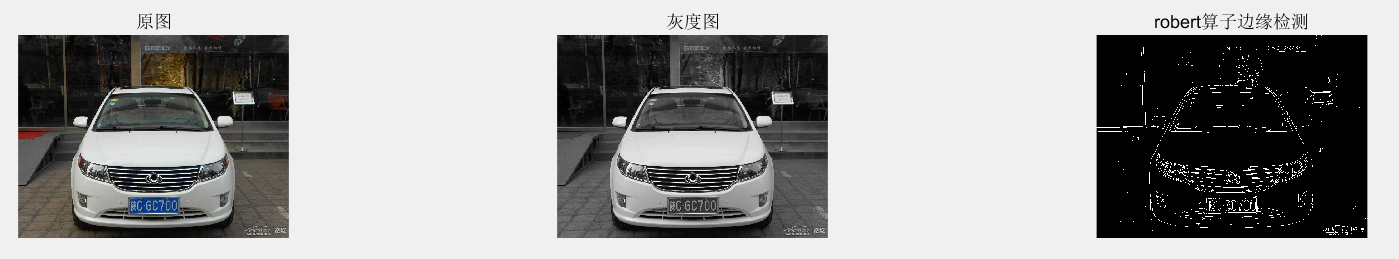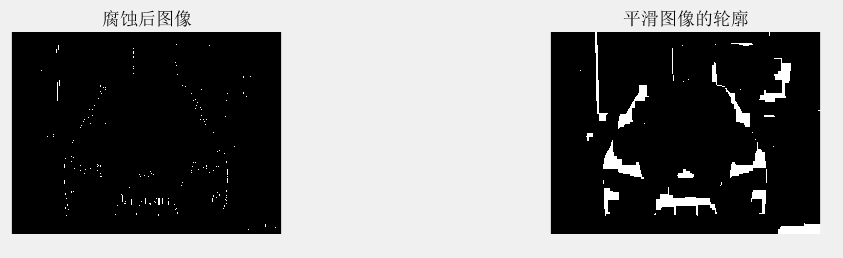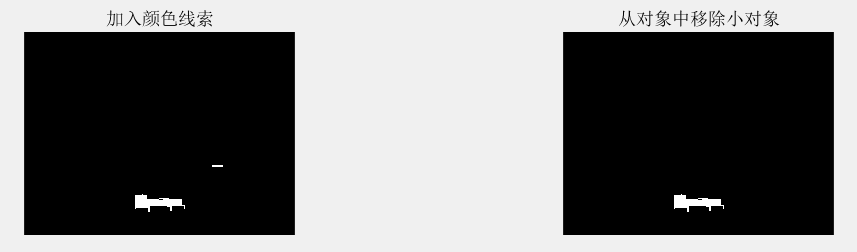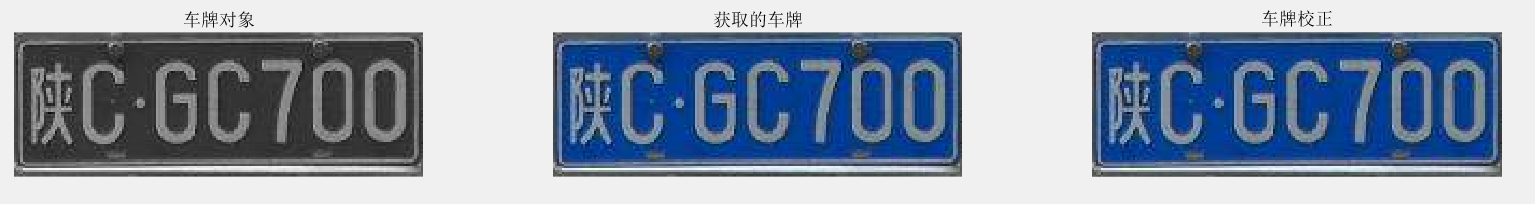# 车牌识别算法实现及其代码实现之一：车牌区域检测

1 首先读入我们要识别的车辆图片，灰度化后，我们使用robert算子对其进行边缘检测；如下图file='2.jpg';
I1=rgb2gray(I);
I2=edge(I1,'roberts',0.08,'both');


2 接着我们对图像进行腐蚀，除去图像中一些不必要的信息，然后我们再对图像进行闭合操作使其区域平滑。se=[1;1;1];
I3=imerode(I2,se);
se=strel('rectangle',[40,40]);%生成一个矩阵
I4=imclose(I3,se);  %闭运算


3 这里我们主要处理小型汽车，即车牌规格为： 440×140 蓝底白字白框线，因此我们获取图像中的蓝色区域，同时腐蚀再闭合，与我们第二步中得到的图像求并，但是我们可以还留存一些小的无用区域，我们还需要对获得的图像进行过滤，去掉小的结构，即得到我们所要获取的车牌区域，如下图I5=removeByColor(I4,I,'12');
I6=bwareaopen(I5,2000);


4 最终获得的车牌区域如下[a,b]=find(I6>0);
I7 = I1(unique(a),unique(b));
I8 = I(unique(a),unique(b),:);


 function im = removeByColor(image,I,removedColor)
% image: 灰度图 x*y
% I: 原始图像 x*y*3
% removedColor:要移除的颜色 1 蓝色、2 黄色、3 白色、4 黑色 默认蓝黄 12
if nargin < 3
removedColor = '12';
end
%大型汽车 440×140 黄底黑字黑框线
%小型汽车 440×140 蓝底白字白框线
diff_Y=0; diff_B=0;

%获取蓝色
Image_B=I;
IR = Image_B(:,:,1);IG = Image_B(:,:,2);IB = Image_B(:,:,3);
XYB=~((IB-IR)>diff_B&(IB-IG)>diff_B);  % 提取蓝色条件是B分量与G、R分量差值大于设定
IR(XYB)=255; IG(XYB)=255; IB(XYB)=255;  % 使得非蓝色区域变为灰色
Image_B(:,:,1)=IR; Image_B(:,:,2)=IG; Image_B(:,:,3)=IB;

Image_B_gray=rgb2gray(Image_B);
stre=[1;1;1];
Image_B_erode=imerode(Image_B_gray,stre);
stre=strel('rectangle',[20,20]);%生成一个矩阵
Image_B_new=imclose(Image_B_erode,stre);  %闭运算

thresh = graythresh(Image_B_new);     %自动确定二值化阈值
bw_Image_B = im2bw(Image_B_new,thresh);       %对图像二值化
im = (~bw_Image_B)&image;
end


figure(1),
subplot(3,3,1),imshow(I),title('原图');hold on;
subplot(3,3,2),imshow(I1),title('灰度图');hold on;
subplot(3,3,3),imshow(I2),title('robert算子边缘检测');hold on;
subplot(3,3,4),imshow(I3),title('腐蚀后图像');hold on;
subplot(3,3,5),imshow(I4),title('平滑图像的轮廓');hold on;
subplot(3,3,6),imshow(I5),title('加入颜色线索');hold on;
subplot(3,3,7),imshow(I6),title('从对象中移除小对象');hold on;
subplot(3,3,8),imshow(I7),title('车牌对象');hold on;
subplot(3,3,9),imshow(I8),title('获取到的车牌');hold on;


©️2019 CSDN 皮肤主题: 编程工作室 设计师: CSDN官方博客